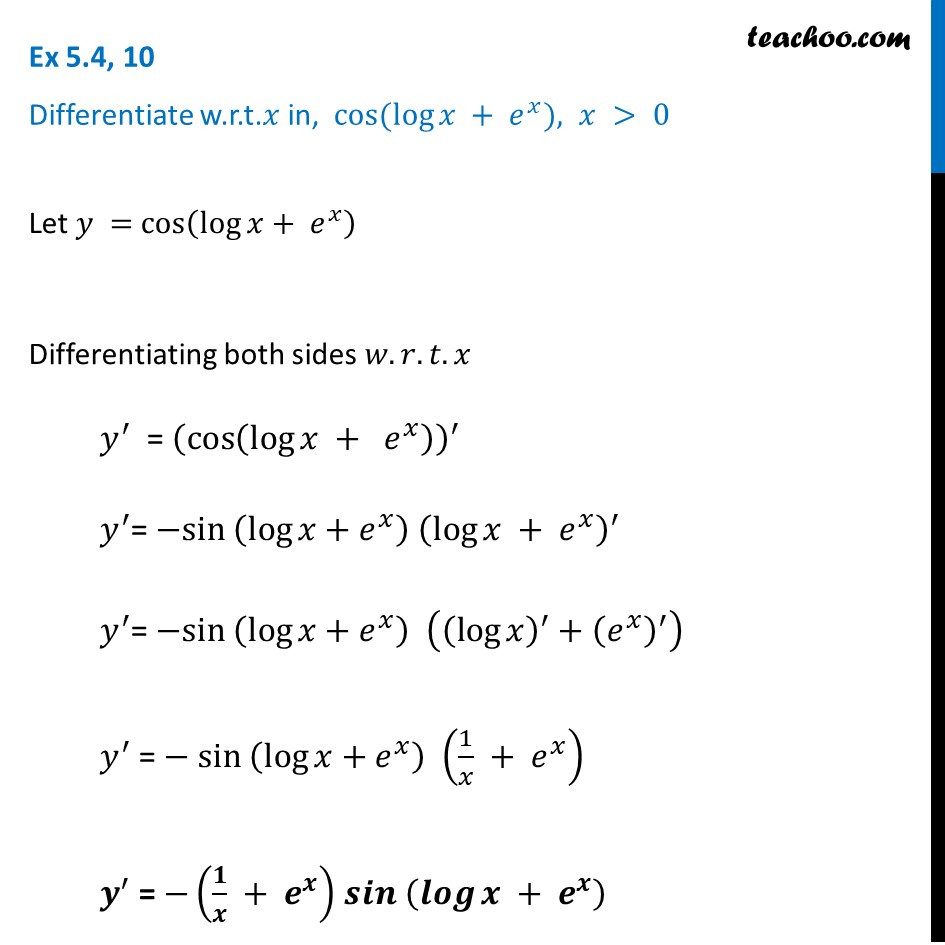Finding derivative of Exponential & logarithm functions

Chapter 5 Class 12 Continuity and Differentiability
Concept wiseIntroducing your new favourite teacher - Teachoo Black, at only ₹83 per month

### Transcript

Ex 5.4, 10 Differentiate w.r.t. 𝑥 in, cos⁡(log⁡𝑥 + 𝑒^𝑥), 𝑥 > 0Let 𝑦 = cos⁡(log⁡𝑥+ 𝑒^𝑥 ) Differentiating both sides 𝑤.𝑟.𝑡.𝑥 𝑦^′ = (cos⁡(log⁡𝑥 + 𝑒^𝑥 ) )^′ 𝑦^′= 〖−sin 〗⁡(log⁡𝑥+𝑒^𝑥 ) (log⁡𝑥 + 𝑒^𝑥 )^′ 𝑦^′= 〖−sin 〗⁡(log⁡𝑥+𝑒^𝑥 ) ((log⁡𝑥 )^′+(𝑒^𝑥 )^′ ) 𝑦^′ = 〖− sin 〗⁡(log⁡𝑥+𝑒^𝑥 ) (1/𝑥 + 𝑒^𝑥 ) 𝒚^′ = −⁡(𝟏/𝒙 + 𝒆^𝒙 ) 𝒔𝒊𝒏 (𝒍𝒐𝒈⁡𝒙 + 𝒆^𝒙 )